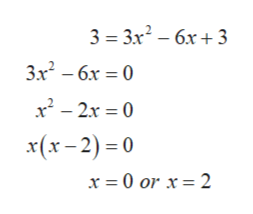# Find equations of both lines that are tangent to the curve y = x3 - 3x2 + 3x - 3 and are parallel to the line 3x - y = 15.

Question

Find equations of both lines that are tangent to the curve y = x3 - 3x2 + 3x - 3 and are parallel to the line 3x - y = 15.

check_circleExpert Solution
Step 1

The given curve is represented by y = x3 - 3x2 + 3x - 3 and are parallel to the line 3x - y = 15.

Slope of line 3x-y=15 is given by,

Step 2

Since the slope of the parallel lines are equal so, the slope of the tangent line will be equal to 3.

Now differentiate the given curve with respect to x in order to find the slope using the curve equation.

Step 3

Now equate both the slope...help_outlineImage Transcriptionclose3 3x2-6x3 3x2-6x 0 x2-2x 0 x(x-2)0 x = 0 or x = 2 fullscreen

### Want to see the full answer?

See Solution

#### Want to see this answer and more?

Solutions are written by subject experts who are available 24/7. Questions are typically answered within 1 hour*

See Solution
*Response times may vary by subject and question
Tagged in

### Derivative